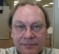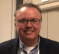## Difference amplitude (x[n] - y[n-1]) quantization in first order iirStarted by 3 years ago14 replieslatest reply 3 years ago255 views

Hi,

I am dealing with the first order iir filter. Formula is y[n] = y[n-1] + alfa*(x[n] - y[n-1]). x[n] is new input sample while y[n] is the output. I quantized the difference amplitude term (x[n] - y[n-1]). I did this with uniform quantization steps. In this way the rate of change is constant if the amplitude difference falls within same quantization step.  However my approach is heuristic. My concern is how can this be theoretically explained and are there real practical examples of this kind of processing? Also are there any stability issues when applying this? In the attachment  is a example of obtained output with this approach. As you can see the output is not converging to the input and this is because of the amplitude difference quantization in iir filter. The use of this is to make this filter more robust to noise that can come from the input signal.

Best Regards

[ - ]Why don't you quantise the full multiplication term alfa*(x[n] - y[n-1]) or even after final addition of y(n-1). Of course you can't allow full bit growth but you will need to truncate result to some suitable resolution that is ok for your case. That point of truncation will have effect on performance.

[ - ]Well these are questions that I would like to learn. Real world examples of when this kind of processing is used.

alfa term is just a constant forgetting rate. It is not something to quantize at least this is what I think.

Also I added the second same plot but with 4-th digit precision on the output signal.

[ - ]I do FPGA platform which is somehow the basis of dsp as well at low level but normally hidden from you in the decimal point representation.

For example assume input and output are 16 bits signed and I choose internal bus as 33 bits.

For an IIR filter with alpha coefficient I will represent alpha on as many bits as I see adequate e.g. I might scale it by 2^16 integer. Then multiply the scaled alpha by the result of y(n-1) - x(n). The difference result is given 33 bits. The multiplication result is given 33 bits. This is going to enter back as y(n-1) later as 33 bits.

The final output is truncated from internal result to 16 bits by discarding 16 LSBs plus one MSB so that I get the original coefficient descaled and so control the gain.

The choice of internal bit width is dependent on input/output dynamic range.

compare this to FPGA based FIR where you can allow full bit growth, or to FFT where internal bit growth is not allowed normally.

[ - ]I'm guessing that alfa < 1, otherwise, there may be uncontrolled bit grow (and a need for saturation logic in the processing path).

Looking at your figure, I see the input (with quantitization noise) has a step change and that the "filtered" output "almost" follows the input after its "settling time". This looks and acts like a weighted filter, maybe only for the simple, bivalue input case.

One other observation is that any differencing operation accentuates noise, so you may need a low pass operation to mitigate noise (even though that adds to it's output delay).

[ - ]alfa = 1.991635132e-4.

Correct alfa is way below 1.‬

[ - ]Hi Mr. Artmez,

Yes it is correct. This is like a weighted filter. You may see how usual simple first order IIR output response compares with this differenced IIR. Blue is the input, red output is obtained with standard IIR, while yellow is with quantized difference approach.

Yes exactly what I would like to discus. So by quantizing this difference x[n] - y[n-1] noise/instability can be introduced? How can this be mitigated since I still would like to have the yellow response but to avoid potential problems.

Best Regards

[ - ]Hi max3031.

I don't know what you mean by quantizing the "difference amplitude term (x[n] - y[n-1])". But I would like to say:

If I'm not mistaken your IIR filter is nothing more than an "exponential averager". (Some people call it a "leaky integrator".) In its traditional implementation your filter is guaranteed to be stable so long as alpha is less than one. That's because the filter's single z-plane pole is located at

z = 1-alpha.

So when alpha < 1, then the z-plane pole will always be inside the z-plane's unit circle.

[ - ]Hi Mr. Lyons,

I will try to explain. I used fixed 0.04 quantization steps

So at first if (abs(x[n] - y[n-1])) < 0.02 step = 0 (just in first case 0.02 is used)

else if ((abs(x[n] - y[n-1]) - 0.02) <= n * quantized_steps) step = alfa * n* quantized_step (where n is the first number that satisfies inequality) and so on

in the end..

if (x > y) then y = y + step

else if (x < y) y = y- step

this is mimicking the exponential filter. Question is weather it is correct to use constant quantization step for this difference or maybe logarithmic? I want to have output like this it is just that I think that something more is needed here from theoretical point in order to work 100% correct.

Best Regards

[ - ]First, we need alfa < 1 for stability.  If alfa > 0 then we have a lowpass characteristic with "dc gain" of unity.

Regarding quantization, another contributor mentions that the quantization is usually performed when the calculated value has to be stored back in memory.  That is, the ALU has a wider wordlength than memory locations do.  That is, the operation is like y[n] = (( y[n-1] + alfa*(x[n] - y[n-1]) ))  where the double parentheses ((....)) refer to quantization.  This operation will be familiar to those in the group that used DSPs like the Motorola 56000 (what a beauty!) where the wordlength for variables was 24 bits whereas the ALU could support intermediate calculation wordlengths of 56 bits.

Denoting the quantization error by e[n], the operation becomes y[n] = y[n-1] + alfa*(x[n] - y[n-1]) + e[n].  Applying the principles of linearity and superposition we can model the impact of quantization, ye[n],  by the difference equation

ye[n] = (1 - alfa)*ye[n-1] + e[n]

Assuming 1 > alfa > 0, this is a lowpass characteristic with "dc gain" equal to (1/alfa).  If alf is close to zero, corresponding to a narrowband lowpass filter then the quantization error experience a very large gain.  Be careful!

[ - ]I have all math capabilities and there is no problem of doing it in double precision.

All that I am concerned with is weather my filter is stable? I divided the difference x[n] - y[n-1] in uniform steps in order to make filter more robust to very tiny noise. Now the key question is on theoretical side weather this approach is correct or certain modifications are necessary?

For example since first order IIR or EWMA has exponential response I am not sure that my solution with uniform step division of difference change is valid. Maybe instead of uniform steps I should take logarithm steps.. Just guessing not sure.

Best

[ - ]Hi max,

I'm not sure what your question has to do with existing IIR or EWMA approaches.  As near as I can figure... you are quantizing a subcomponent of your filter calculation - the difference - to desensitize your output to input noise.  If you do that, it is now no longer a linear filter.  So, evaluating it with linear filter theory is not reasonable.

Is it stable?  Well, in a BIBO sense, sure.  ...but I don't think that looking at it on the complex plane will get you anywhere.

Question #1...

You posted a figure with your input, a red line, and a yellow line.  You said that you wanted the yellow line.  The yellow line had steady state error.  Why do you want an estimate with sustained steady state error?

Question #2...

Why not just filter the input data to reduce the noise and then use the unmodified averager?

I'm not saying that you can't fuzzify the difference in your calculation as you have.  ...but - if you do - the normal methods of evaluation no longer necessarily apply.

Dan

[ - ]Hi Mr. Dan,

Yes I see your point. However this looks like it was modeled with EWMA or first order iir. The way it is done is non-linear for sure. Reason why was to robustly remove noise. My heuristics by no-means is good approach that is why I am looking for the ways to obtain very close response (yellow) but with non-linear background in math and signal processing theoretical concept. Not heuristics that I did.

If you know such methods please let me know to further discus.

Best

[ - ]Hi max,

I understand better your question.  I made reference to "fuzzifying" your difference.  In an essence, you are applying a hard threshold to your difference by quantizing.  A not-so-fuzzy fuzzification.  So... maybe you could make your transitions non-binary.  If so, you are in the realm of fuzzy logic.

If you want to maintain a separation of your bins, then (in my opinion) you are in the realm of gravitational searches or attractors.  You could imagine the possible forms of your IIR filter being discrete locations on the complex plane whereby - if your difference put you close - your IIR coefficient would gravitate to the nearest allowable quantized result.  I would look into those areas to establish formalism.

Best,

Dan

[ - ]